xOracle machineEncyclopedia
In complexity theory
Computational complexity theory
Computational complexity theory is a branch of the theory of computation in theoretical computer science and mathematics that focuses on classifying computational problems according to their inherent difficulty, and relating those classes to each other...

and computability theory, an oracle machine is an abstract machine
Abstract machine
An abstract machine, also called an abstract computer, is a theoretical model of a computer hardware or software system used in automata theory...

used to study decision problem
Decision problem
In computability theory and computational complexity theory, a decision problem is a question in some formal system with a yes-or-no answer, depending on the values of some input parameters. For example, the problem "given two numbers x and y, does x evenly divide y?" is a decision problem...

s. It can be visualized as a Turing machine
Turing machine
A Turing machine is a theoretical device that manipulates symbols on a strip of tape according to a table of rules. Despite its simplicity, a Turing machine can be adapted to simulate the logic of any computer algorithm, and is particularly useful in explaining the functions of a CPU inside a...

with a black box, called an oracle
Oracle
In Classical Antiquity, an oracle was a person or agency considered to be a source of wise counsel or prophetic predictions or precognition of the future, inspired by the gods. As such it is a form of divination....

, which is able to decide certain decision problems in a single operation. The problem can be of any complexity class
Complexity class
In computational complexity theory, a complexity class is a set of problems of related resource-based complexity. A typical complexity class has a definition of the form:...

. Even undecidable problem
Undecidable problem
In computability theory and computational complexity theory, an undecidable problem is a decision problem for which it is impossible to construct a single algorithm that always leads to a correct yes-or-no answer....

s, like the halting problem
Halting problem
In computability theory, the halting problem can be stated as follows: Given a description of a computer program, decide whether the program finishes running or continues to run forever...

, can be used.

## Definition

An oracle machine is a Turing machine
Turing machine
A Turing machine is a theoretical device that manipulates symbols on a strip of tape according to a table of rules. Despite its simplicity, a Turing machine can be adapted to simulate the logic of any computer algorithm, and is particularly useful in explaining the functions of a CPU inside a...

connected to an oracle. The oracle, in this context, is thought of as an entity capable of answering some collection of questions, and usually represented as some subset A of the natural numbers. Intuitively then, the oracle machine can perform all of the usual operations of a Turing machine, and can also query the oracle for an answer to a specific question of the form "is x in A?"

The definition given here is one of several possible oracle machine definitions. All these definitions are equivalent, as they agree on which specific functions f can be computed by an oracle machine with oracle A.

An oracle machine, like a Turing machine, includes:
• a work tape: a sequence of cells without beginning or end, each of which may contain a B (for blank) or a 1;
• a read/write head, which rests on a single cell of the work tape and can read the data there, write new data, and move left or right along the tape;
• a control mechanism, which can be in one of a finite number of states, and which will perform different actions (reading data, writing data, moving the control mechanism, and changing states) depending on the current state and the data being read.

In addition to these components, an oracle machine also includes:
• an oracle tape, on which an infinite sequence of B's and 1's is printed, corresponding to the characteristic function of the oracle set A;
• an oracle head, which (like the read/write head) can move left or right along the oracle tape reading data, but which cannot write.

### Formal definition

An oracle Turing machine is a 4-tuple
Tuple
In mathematics and computer science, a tuple is an ordered list of elements. In set theory, an n-tuple is a sequence of n elements, where n is a positive integer. There is also one 0-tuple, an empty sequence. An n-tuple is defined inductively using the construction of an ordered pair...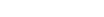where
•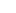is a finite set of states
•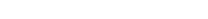is a partial function
Partial function
In mathematics, a partial function from X to Y is a function ƒ: X' → Y, where X' is a subset of X. It generalizes the concept of a function by not forcing f to map every element of X to an element of Y . If X' = X, then ƒ is called a total function and is equivalent to a function...

called the transition function, where L is left shift, R is right shift.
•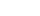is the initial state
•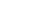is the set of halting states.

The oracle machine is initialized with the work tape containing some input with finitely many 1's and the rest of the tape blank, the oracle tape containing the characteristic function of the oracle, A, and the Turing machine in state q0 with read/write head reading the first nonblank cell of the work tape, and oracle head reading the cell of the oracle tape which corresponds to. Thereafter it operates according to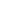: if the Turing machine is currently in state q, the read/write head is reading a symbol S1, and the oracle head is reading S2, then if, the machine enters state, the read/write head writes the symbol S1' in place of S1, and then the read/write head moves 1 cell in direction D1 and the oracle head moves one cell in direction D2. At this point if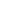is a halting state, the machine halts, otherwise it repeats this same procedure.

Turing machines can compute functions as follows: if f is a function that takes natural numbers to natural numbers, MA is a Turing machine with oracle A, and whenever MA is initialized with the work tape consisting of n+1 consecutive 1's (and blank elsewhere) MA eventually halts with f(n) 1's on the tape, then MA is said to compute the function f. A similar definition can be made for functions of more than one variable, or partial functions.

If there is an oracle machine M that computes a function f with oracle A, f is said to be A-computable. If f is the characteristic function of a set B, B is also said to be A-computable, and M is said to be a Turing reduction
Turing reduction
In computability theory, a Turing reduction from a problem A to a problem B, named after Alan Turing, is a reduction which solves A, assuming B is already known . It can be understood as an algorithm that could be used to solve A if it had available to it a subroutine for solving B...

from B to A.

## Complexity classes of oracle machines

The complexity class
Complexity class
In computational complexity theory, a complexity class is a set of problems of related resource-based complexity. A typical complexity class has a definition of the form:...

of decision problem
Decision problem
In computability theory and computational complexity theory, a decision problem is a question in some formal system with a yes-or-no answer, depending on the values of some input parameters. For example, the problem "given two numbers x and y, does x evenly divide y?" is a decision problem...

s solvable by an algorithm in class A with an oracle for a language L is called AL. For example, PSAT is the class of problems solvable in polynomial time by a deterministic Turing machine with an oracle for the Boolean satisfiability problem
Boolean satisfiability problem
In computer science, satisfiability is the problem of determining if the variables of a given Boolean formula can be assigned in such a way as to make the formula evaluate to TRUE...

. The notation AB can be extended to a set of languages B (or a complexity class B), by using the following definition:When a language L is complete
Complete (complexity)
In computational complexity theory, a computational problem is complete for a complexity class if it is, in a formal sense, one of the "hardest" or "most expressive" problems in the complexity class...

for some class B, then AL=AB provided that machines in A can execute reductions used in the completeness definition of class B. In particular, since SAT is NP-complete
NP-complete
In computational complexity theory, the complexity class NP-complete is a class of decision problems. A decision problem L is NP-complete if it is in the set of NP problems so that any given solution to the decision problem can be verified in polynomial time, and also in the set of NP-hard...

with respect to polynomial time reductions, PSAT=PNP. However, if A = DLOGTIME
DLOGTIME
DLOGTIME is the complexity class of all computational problems solvable in a logarithmic amount of computation time on a deterministic Turing machine. It is the smallest nontrivial class using the resource of deterministic time...

, then ASAT may not equal ANP.

It is obvious that NP ⊆ PNP, but the question of whether NPNP, PNP, NP, and P are equal remains tentative at best. It is believed they are different, and this leads to the definition of the polynomial hierarchy
Polynomial hierarchy
In computational complexity theory, the polynomial hierarchy is a hierarchy of complexity classes that generalize the classes P, NP and co-NP to oracle machines...

.

Oracle machines are useful for investigating the relationship between complexity classes P and NP, by considering the relationship between PA and NPA for an oracle A. In particular, it has been shown there exist languages A and B such that PA=NPA and PB≠NPB.
The fact the P = NP question relativizes both ways is taken as evidence that answering this question is difficult, because a proof technique that relativizes (i.e., unaffected by the addition of an oracle) will not answer the P = NP question. Most proof techniques relativize.

It is interesting to consider the case where an oracle is chosen randomly from among all possible oracles (an infinite set). It has been shown in this case, then with probability 1, PA≠NPA. When a question is true for almost all oracles, it is said to be true for a random oracle. This choice of terminology is justified by the fact random oracles support a statement with probability 0 or 1 only. (This follows from Kolmogorov's zero one law.) This is taken as evidence P≠NP. A statement may be true for a random oracle
Random oracle
In cryptography, a random oracle is an oracle that responds to every query with a random response chosen uniformly from its output domain, except that for any specific query, it responds the same way every time it receives that query...

and false for ordinary Turing machines at the same time; for example for oracles A, IPA≠PSPACEA, while IP
IP (complexity)
In computational complexity theory, the class IP is the class of problems solvable by an interactive proof system. The concept of an interactive proof system was first introduced by Shafi Goldwasser, Silvio Micali, and Charles Rackoff in 1985...

= PSPACE
PSPACE
In computational complexity theory, PSPACE is the set of all decision problems which can be solved by a Turing machine using a polynomial amount of space.- Formal definition :...

.

## Oracles and halting problems

It is possible to posit the existence of an oracle which computes a non-computable function, such as the answer to the halting problem
Halting problem
In computability theory, the halting problem can be stated as follows: Given a description of a computer program, decide whether the program finishes running or continues to run forever...

or some equivalent. A machine with an oracle of this sort is a hypercomputer.

Interestingly, the halting paradox still applies to such machines; although they determine whether particular Turing machines will halt on particular inputs, they cannot determine, in general, if machines equivalent to themselves will halt. This fact creates a hierarchy of machines, called the arithmetical hierarchy
Arithmetical hierarchy
In mathematical logic, the arithmetical hierarchy, arithmetic hierarchy or Kleene-Mostowski hierarchy classifies certain sets based on the complexity of formulas that define them...

, each with a more powerful halting oracle and an even harder halting problem.

## Applications to cryptography

In cryptography
Cryptography
Cryptography is the practice and study of techniques for secure communication in the presence of third parties...

, oracles are used to make arguments for the security of cryptographic protocols where a hash function
Cryptographic hash function
A cryptographic hash function is a deterministic procedure that takes an arbitrary block of data and returns a fixed-size bit string, the hash value, such that an accidental or intentional change to the data will change the hash value...

is used. A security reduction
Provable security
In cryptography, a system has provable security if its security requirements can be stated formally in an adversarial model, as opposed to heuristically, with clear assumptions that the adversary has access to the system as well as enough computational resources...

for the protocol is given in the case where, instead of a hash function, a random oracle
Random oracle
In cryptography, a random oracle is an oracle that responds to every query with a random response chosen uniformly from its output domain, except that for any specific query, it responds the same way every time it receives that query...

answers each query but consistently; the oracle assumes to be available to all parties including the attacker, as the hash function is. Such a proof shows unless the attacker solves the hard problem at the heart of the security reduction, they must make use of some interesting property of the hash function to break the protocol; they cannot treat the hash function as a black box (i.e., as a random oracle).

• Alan Turing, Systems of logic based on ordinals, Proc. London math. soc., 45, 1939
• C. Papadimitriou. Computational Complexity. Addison-Wesley, 1994. Section 14.3: Oracles, pp. 339–343.
• Michael Sipser
Michael Sipser
Michael Fredric Sipser is a professor of Applied Mathematics in the Theory of Computation Group at the Massachusetts Institute of Technology. He received his Ph.D. in 1980 from the University of California, Berkeley under the direction of Manuel Blum....

. Introduction to the Theory of Computation. PWS Publishing, 1997. ISBN 0-534-94728-X. Section 9.2: Relativization, pp.318–321.
• Martin Davis
Martin Davis
Martin David Davis, is an American mathematician, known for his work on Hilbert's tenth problem . He received his Ph.D. from Princeton University in 1950, where his adviser was Alonzo Church . He is Professor Emeritus at New York University. He is the co-inventor of the Davis-Putnam and the DPLL...

, editor: The Undecidable, Basic Papers on Undecidable Propositions, Unsolvable Problems And Computable Functions, Raven Press, New York, 1965. Turing's paper is in this volume. Papers include those by Godel, Church, Rosser, Kleene, and Post.